## Microsoft Office 2003

#### Course at a glance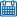Release date 10/25/2005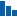Level Beginner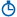Runtime 16h 5m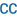Closed captioning N/A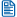Transcript N/A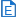eBooks / courseware Included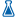Hands-on labs N/A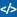Sample code Included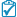Exams Included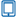Platform Internet Explorer Only##### Enterprise Solutions

Need reporting, custom learning tracks, or SCORM? Learn More

#### Course description

Join AppDev expert Jan Fransen as she teaches the effective use of Microsoft Office 2003. Maximize your productivity with this comprehensive training suite. This suite takes you step-by-step through using Microsoft Word, Excel, PowerPoint and Outlook.

#### Meet the expertJan Fransen has been working to educate people in the best ways to use Microsoft products for more than 15 years. She's written both data-based and document-based applications for large and small companies and has helped hundreds of Office users and developers get started on the right foot through public and onsite training classes. As a writer, Jan has developed training courseware, contributed to books on Microsoft Office, written white papers for publication on MSDN, and created samples designed to help developers get up to speed quickly on new Microsoft products and features.

#### Word 2003 - 1

##### Working with Word (17:06)
• Introduction (00:28)
• Starting Word (00:50)
• Quick Overview of Features (03:50)
• Menu and Toolbar Options (03:37)
• Using Task Panes (00:58)
• Opening a Document (02:05)
• Managing Open Documents (02:49)
• Using Word Help (02:09)
• Summary (00:17)
##### Working with Text (10:49)
• Introduction (00:31)
• Positioning Insertion Point (02:07)
• Selecting Text (01:01)
• Insert, Replace, Delete text (00:53)
• Changing Character Attributes (01:38)
• Styles and Formatting (01:10)
• Using AutoCorrect (00:41)
• Replace Text As You Type (00:32)
• Built In Replace Text Entries (01:19)
• Using AutoComplete (00:36)
• Summary (00:15)
##### Working with Documents (03:18)
• Introduction (00:18)
• Creating a Document (01:00)
• Saving a Document (00:43)
• Closing a Document (00:05)
• Close Multiple Documents (00:31)
• Exiting Word (00:11)
• Summary (00:26)
##### Revising Dcouments (11:16)
• Introduction (00:42)
• Copy, Cut, and Move Text (04:01)
• Using the Office Clipboard (02:27)
• Using Undo and Redo (02:09)
• Using Repeat (01:27)
• Summary (00:28)
##### Using Document Tools (09:19)
• Introduction (00:30)
• Check Spelling and Grammar (00:51)
• Spelling/Grammar Options (03:35)
• Spelling/Grammar Shortcuts (01:30)
• Using the Thesaurus (00:46)
• Research Task Pane (01:10)
• Synonyms (00:37)
• Summary (00:15)
##### Formatting Paragraphs (08:36)
• Introduction (00:24)
• Changing Line Spacing (01:56)
• Aligning Text (01:37)
• Indenting Text (00:45)
• Setting Tabs (00:22)
• Show/Hide button (00:26)
• Tabs Menu (02:48)
• Summary (00:16)

#### Word 2003 - 2

##### Working with Pages and Views (11:44)
• Introduction (00:24)
• Working with Views (00:50)
• Web Layout View (00:14)
• Print Layout View (00:19)
• Hide/Show White Space (00:37)
• Reading Layout (00:21)
• Outline View (00:54)
• Inserting Page Breaks (02:09)
• Using Click and Type (01:47)
• Changing Margins (00:13)
• Page Setup Dialog (01:12)
• Creating Page Numbers (02:11)
• Summary (00:28)
##### Printing Documents (08:14)
• Introduction (00:22)
• Using Print Preview (00:46)
• Zoom in/out on Print Preview (00:51)
• Change Zoom Magnification (00:19)
• Multiple Pages Toolbar Button (00:38)
• Printing a Document (00:22)
• Print Dialog (01:32)
• Creating/Printing Envelopes (02:56)
• Summary (00:24)
##### Working with Tables (16:45)
• Introduction (00:33)
• Creating a Table (01:02)
• Use Draw Table Tool (00:52)
• Enter Text into a table (02:18)
• Select Parts of a Table (00:49)
• Insert Columns, Rows, Cells (02:50)
• Adjust Columns, Rows, Cells (00:52)
• Using Table Properties Dialog (01:51)
• Distribute Rows Evenly Tool (02:26)
• Using the AutoFit Tool (00:28)
• Delete Tables/Cols/Rows/Cells (01:05)
• Copy, Move, Resize Tables (01:10)
• Summary (00:23)
##### Formatting Tables (25:30)
• Introduction (00:30)
• Sorting Tables (02:25)
• Apply Borders and Shading (01:47)
• Merging and Splitting Cells (01:46)
• Changing Text Alignment (00:32)
• Rotating Text in a Table (00:36)
• Using AutoFormat in a Table (03:04)
• Formulas and Functions (01:18)
• Add a Formula to a Table Cell (03:29)
• Table and Cell Properties (01:57)
• Change Table Borders/Shade (01:50)
• Change Cell Margins (02:34)
• Working with Nested Tables (03:18)
• Summary (00:16)
##### Using Special Text Features (14:30)
• Introduction (00:41)
• Inserting Symbols (01:57)
• Create AutoText (00:43)
• Insert AutoText (00:46)
• Insert an AutoText Closing (01:08)
• Change AutoText (00:48)
• AutoText Toolbar (00:25)
• Remove AutoText (00:25)
• Replacing Document Text (04:48)
• Using Navigational Tools (00:57)
• Select Browse Object (00:33)
• Browse by Page (00:17)
• Browse by Graphics (00:31)
• Summary (00:23)

#### Word 2003 - 3

##### Borders and Graphics (08:07)
• Introduction (00:30)
• Apply Page Borders (01:46)
• Apply Borders and Shading (01:26)
• Draw/Change Lines/Objects (02:48)
• Using the Drawing Grid (01:13)
• Summary (00:21)
##### Working with Sections (11:11)
• Introduction (00:25)
• Create a Section (01:44)
• Paper Size and Orientation (01:45)
• Create a Title Page (00:44)
• Change Vertical Alignment (00:37)
• Create Headers and Footers (02:46)
• Add Columns to a Report (01:22)
• Add a Column Break (00:23)
• Add a Continuous Break (01:01)
• Summary (00:17)
##### Working with Special Features (11:43)
• Introduction (00:27)
• Bulleted and Numbered Lists (01:41)
• Modify the Bullet Markers (03:19)
• Numbered Outline (01:47)
• Footnotes and Endnotes (00:53)
• Adding a Footnote (01:32)
• Modifying a Footnote (00:53)
• Change Footnote Separator (00:49)
• Summary (00:17)
##### Creating Merge Documents (13:50)
• Introduction (00:32)
• Define Merge Terms (00:41)
• Creating Merge Documents (00:31)
• Run an Exisitng Merge (02:41)
• Create a Mail Merge (00:45)
• Work with the Data Source (01:56)
• Work with the Main Document (00:14)
• Add the Merge Fields (01:29)
• Preview the Letter (00:51)
• Complete the Merge (00:23)
• Using the Mail Merge Toolbar (03:23)
• Summary (00:17)
##### Edit Merge Documents (09:02)
• Introduction (00:23)
• Modify Records in Data Source (02:18)
• Customize Data Source (02:10)
• Create Mailing Labels (02:23)
• Filter/Sort the Data Source (01:22)
• Summary (00:24)
##### Styles and Themes (13:33)
• Introduction (00:23)
• Create a Style (02:19)
• Use a Style (01:57)
• Edit a Style (00:48)
• Merge Styles (02:38)
• Use the Style Gallery (01:06)
• Create Styles Automatically (00:58)
• Change Styles by Example (01:51)
• Apply a Theme (01:06)
• Summary (00:22)

#### Word 2003 - 4

##### Working with Templates (07:32)
• Introduction (00:27)
• Using Templates (01:42)
• Creating a Template (02:51)
• Editing a Template (02:01)
• Summary (00:29)
##### Working with Fields (16:36)
• Introduction (00:37)
• Defining Fields (00:32)
• Defining Field Components (00:18)
• Inserting Fields (01:28)
• Displaying Field Codes (01:15)
• Updating a Field (01:23)
• Editing a Field (01:03)
• Creating an Index (02:39)
• Using IF Field in Mail Merge (01:46)
• Using a FILLIN Field (02:43)
• Summary (00:30)
##### Working with Macros (08:56)
• Introduction (00:28)
• Recording a Macro (02:18)
• Running a Macro (01:05)
• Editing a Macro (01:29)
• Assign Macro to Shortcut Key (01:11)
• Assign Macro to Menu/Toolbar (01:00)
• Deleting a Macro (00:58)
• Summary (00:25)
##### Creating Forms (06:52)
• Introduction (00:26)
• Placing a Form Field (01:35)
• Modify Form Field Properties (01:43)
• Adding Help Text to A Field (00:48)
• Protecting a Form (00:41)
• Using a Form (01:17)
• Summary (00:20)
##### Working with Graphics (15:42)
• Introduction (00:25)
• Inserting Clip Art (02:11)
• Insert a Picture From a File (00:47)
• Wrap Text Around Graphics (01:42)
• Select/Position Graphics (01:17)
• Size and Crop Graphics (02:18)
• Drawing and Picture Toolbars (00:28)
• Align/Distribute Graphics (02:31)
• Create Linked Text Boxes (02:32)
• Create Watermarks (01:05)
• Summary (00:22)
##### Worksheets, Charts, Diagrams (09:47)
• Introduction (00:43)
• Inserting Excel Data (01:27)
• Creating a Chart (01:56)
• Modifying a Chart (01:51)
• Import Excel Data (01:11)
• Import an Excel Chart (01:16)
• Insert Diagrams (00:55)
• Summary (00:25)
##### Collaborative Tools (10:23)
• Introduction (00:24)
• Configure Docs. for Tracking (01:01)
• Protect the Document (01:01)
• Track Document Changes (01:38)
• Accept/Reject Tracked Change (02:44)
• Save Document Versions (01:07)
• Merge Document Changes (00:20)
• Merge Options (00:17)
• Compare Versions (00:35)
• Merge Changes Into 1 Doc. (00:44)
• Summary (00:27)

#### Excel 2003 - 1

##### Getting Started (16:52)
• Introduction (00:34)
• Starting Excel (00:41)
• The Display Screen (03:05)
• Change Menu Options (01:20)
• Change Toolbar Options (02:03)
• Using Task Panes (01:09)
• Opening a Workbook (00:35)
• Working with the Open Dialog (01:41)
• Managing Open Workbooks (00:26)
• Workbooks in Task Bar (00:50)
• Using Smart Tags (02:03)
• Using Excel Help (01:56)
• Summary (00:24)
##### Entering Data (15:00)
• Introduction (00:30)
• Moving the Cell Pointer (01:12)
• Use GoTo Dialog (00:42)
• Selecting a Range of Cells (01:59)
• Entering Constant Values (03:52)
• Saving a Workbook (01:09)
• Editing/Clearing Cell Contents (03:10)
• Working with Undo and Redo (01:09)
• Closing a Workbook (00:28)
• Exiting Excel (00:16)
• Summary (00:27)
##### Using Formulas (14:46)
• Introduction (00:38)
• Entering Formulas (02:12)
• Use the SUM Function (02:21)
• Auto. Sum Columns/Rows (01:53)
• Use Statistical Functions (02:24)
• Work with the Range Finder (00:51)
• Use Formula Error Checking (03:50)
• Summary (00:35)
##### Constant Values and Formulas (13:44)
• Introduction (01:08)
• Copy/Paste Contents of Cells (03:40)
• Move Contents of Cells (01:26)
• Use Office Clipboard (02:58)
• Use AutoFill (02:05)
• Absolute Cell References (01:58)
• Summary (00:25)
##### Formatting Worksheets (08:07)
• Introduction (00:26)
• Formatting Numbers (02:13)
• Changing the Font Format (01:20)
• Aligning Cell Contents (00:30)
• Rotate Text (00:35)
• Merging Cells (01:14)
• Adding Borders (00:37)
• Using AutoFormat (00:55)
• Summary (00:14)
##### Modify Columns and Rows (09:12)
• Introduction (00:23)
• Change Column Width (01:25)
• Change Row Height (00:28)
• Use AutoFit (00:54)
• Insert/Delete Columns/Rows (02:46)
• Hide Columns/Rows (01:15)
• Use Custom Worksheet Views (01:45)
• Summary (00:14)

#### Excel 2003 - 2

##### Editing Workbooks (04:49)
• Introduction (00:22)
• Work with AutoCorrect (01:37)
• Check Spelling (01:06)
• Use Find and Replace (01:25)
• Summary (00:18)
##### Printing Worksheets (09:24)
• Introduction (00:26)
• Use Print Preview (01:10)
• Adjust Margins/Column Widths (00:54)
• Use Page Break Preview (01:57)
• Use Page Setup (02:01)
• Create a Header/Footer (01:52)
• Print a Worksheet (00:45)
• Summary (00:15)
##### Working with Worksheets (20:26)
• Introduction (00:34)
• View Worksheets (01:17)
• Rename Worksheets (00:34)
• Color-Code Worksheets (00:43)
• Create A Group (01:07)
• Edit a Group (02:01)
• Copy/Move Worksheets (02:14)
• Insert a New Worksheet (00:52)
• Create Linked Formulas (01:56)
• Paste Special (00:24)
• Paste Links (00:50)
• Use 3-D References (01:02)
• Use SUM Function (01:17)
• Protect Workbooks (01:53)
• Protect Worksheets (00:12)
• Unlock Cells (03:00)
• Summary (00:23)
##### Working with Functions (20:03)
• Introduction (00:39)
• Create/Use Named Ranges (03:16)
• Go To dialog box (00:17)
• Create a Relative Name (03:30)
• Use Custom Zoom (01:10)
• Using Functions (00:33)
• Insert Function dialog box (02:32)
• Using the Function List (01:11)
• Use Financial Functions (04:37)
• Use Date Functions (01:44)
• Summary (00:29)
##### Formatting and Data Validation (20:39)
• Introduction (00:34)
• Conditional Formatting (02:17)
• Adding Multiple Conditions (02:19)
• Go To / Special dialog (00:33)
• Using Data Validation (04:08)
• Formula Auditing Toolbar (04:27)
• Setting Up a Custom Format (02:11)
• Auditing Tools (01:46)
• Error Checking Tools (02:00)
• Summary (00:18)

#### Excel 2003 - 3

##### Creating Charts (17:27)
• Introduction (00:25)
• Use the Chart Wizard (03:01)
• Modifying Charts (01:01)
• Chart Toolbar (01:34)
• Changing the Chart Type (01:38)
• Change Chart Location/Size (01:11)
• Changing Chart Options (02:47)
• Add/Remove Data Series (03:16)
• Changing Worksheet Data (01:07)
• Using Range Finder w/ Charts (00:55)
• Summary (00:28)
##### Working with Charts (14:04)
• Introduction (00:20)
• Using Text Boxes (03:10)
• Formatting Chart Elements (02:34)
• Formatting Chart Text (02:38)
• Formatting Chart Objects (02:19)
• Printing Charts (02:35)
• Summary (00:23)
##### Creating Advanced Charts (11:06)
• Introduction (00:19)
• Work with 3-D Charts (02:30)
• Create a Combination Chart (03:26)
• Create Complex Pie Charts (02:20)
• Create a Pictograph (02:09)
• Summary (00:19)
##### Using List Features (12:10)
• Introduction (00:40)
• Create a List (01:16)
• Using AutoComplete (01:59)
• Sorting a List (01:18)
• Using the Data Form (02:15)
• Filtering a List (04:25)
• Summary (00:14)
##### Using Styles and Templates (13:52)
• Introduction (00:41)
• Create and Use Styles (04:20)
• Merge Styles (01:50)
• Using Templates (02:25)
• Create New Templates (03:59)
• Summary (00:34)
##### Advanced Functions (20:52)
• Introduction (00:37)
• Work with the IF Function (03:48)
• IF with Multiple Conditions (05:02)
• Table Lookup Functions (04:15)
• Text Functions (03:18)
• Data Tables (00:46)
• One-Input Data Tables (01:32)
• Two-Input Data Tables (01:08)
• Summary (00:22)

#### Excel 2003 - 4

##### Analyzing Data (18:31)
• Introduction (00:23)
• Using Automatic Outlining (02:07)
• Consolidating Information (02:47)
• Create Links to Source Data (01:11)
• Inserting Subtotals (01:13)
• Inserting Averages (00:53)
• Using Visible Cells Only (01:36)
• Using Custom AutoFilter (01:25)
• Creating an Advanced Filter (03:45)
• Using Database Functions (02:43)
• Summary (00:24)
##### Using PivotTables (07:06)
• Introduction (00:36)
• PivotTable/PivotChart Wizard (01:16)
• Laying Out a PivotTable (00:49)
• Modifying a PivotTable (01:26)
• Work with Page Fields (01:25)
• Refresh a PivotTable (00:54)
• Summary (00:37)
##### Enhance PivotTables (13:40)
• Introduction (00:20)
• Formatting a PivotTable (02:15)
• Work with Summary Functions (03:41)
• Sort Items in a PivotTable (01:03)
• Group Data in a PivotTable (01:09)
• Use AutoShow in a PivotTable (00:32)
• Create a Calculated Field (00:49)
• Create a Calculated Item (00:50)
• Create Charts from P.T. (02:40)
• Summary (00:16)
##### Data Analysis Tools (10:29)
• Introduction (00:22)
• Creating Scenarios (03:38)
• Work with Scenarios (01:14)
• Using Goal Seek (01:41)
• Understanding Solver (00:43)
• Using Solver (02:29)
• Summary (00:19)
##### Working with Macros (07:27)
• Introduction (00:27)
• Recording a Macro (03:24)
• Executing a Macro (00:56)
• Create Macro Buttons (02:06)
• Summary (00:33)
##### Using Special Features (07:24)
• Introduction (00:37)
• Import/Export Data (03:01)
• Text Import Wizard (01:15)
• Work with Hypertext Links (02:14)
• Summary (00:15)
##### Using Shared Workbook Feat. (09:18)
• Introduction (00:42)
• Configuring a Workbook (01:00)
• Highlight Tracked Changes (00:42)
• Working in a Shared Workbook (00:41)
• Add a Comment (00:59)
• Remove a Comment (00:19)
• Review Changes (00:50)
• Accept/Reject Changes (01:15)
• Remove from Shared Use (00:25)
• Merging Workbooks (01:44)
• Summary (00:37)

#### Outlook - 1

##### Getting Started (10:12)
• Introduction (00:33)
• Starting Outlook (01:08)
• Understand the Display Screen (01:43)
• Changing Menu Options (00:08)
• Using Personalized Menus (00:48)
• Using Toolbars (01:24)
• Using the Navigation Pane (01:42)
• Use/Customize Outlook Today (02:16)
• Summary (00:25)
##### Working with the Inbox (21:39)
• Introduction (00:17)
• Create/Send Messages (02:37)
• Add Options to a Message (03:04)
• Using the Reading Pane (01:02)
• Open/Read/Print Messages (02:16)
• Reply To/Forward Messages (02:24)
• Attach a File to a Message (01:33)
• Working with Attachments (01:09)
• Working with Hyperlinks (02:34)
• Office Clipboard Task Pane (03:44)
• Smart Tag Actions Button (00:22)
• Summary (00:31)
##### Using Folders to Manage Mail (08:05)
• Introduction (00:25)
• Working with Folders (01:44)
• Creating a Folder (01:10)
• Moving Messages to a Folder (00:26)
• Using the Drafts Folder (01:40)
• Favorites Folder Pane (01:25)
• Using Public Folders (00:35)
• Summary (00:37)
##### Using Special Mail Features (23:23)
• Introduction (00:29)
• Create/Use Distribution Lists (02:53)
• Use the Organizer Pane (00:46)
• Managing Junk E-Mail (01:37)
• Using the Rules Wizard (02:28)
• Working with Rules (01:24)
• Use the Out of Office Assistant (02:41)
• Creating a Signature (02:12)
• Create/Use Delegates (03:55)
• Use/Track Voting Buttons (03:11)
• Clean Up Your Mailbox (01:13)
• Summary (00:28)

#### Outlook - 2

##### Working with Calendars (13:58)
• Introduction (00:31)
• Using the Calendar (01:29)
• Create Appts. and Events (02:56)
• Create a Recurring Appt. (01:39)
• Modifying Appts./Events (01:47)
• Copy/Move Calendar Items (00:52)
• View Other Users" Calendars (02:18)
• Create a Group Schedule (01:08)
• Summary (00:27)
##### Working with Meetings (08:57)
• Introduction (00:26)
• Creating Meetings (03:49)
• Respond to a Meeting Request (02:32)
• Modifying a Meeting (00:37)
• Deleting a Meeting (01:14)
• Summary (00:18)
##### Working with Contacts (08:42)
• Introduction (00:26)
• Adding a Contact (03:33)
• Update/Delete a Contact (01:00)
• Create Msgs. Using Contact (01:13)
• Working with Categories (02:03)
• Summary (00:25)
##### Using Tasks (07:12)
• Introduction (00:31)
• Create a Task (02:09)
• Modify Tasks (00:44)
• Assign Tasks (02:46)
• Delete Tasks (00:39)
• Summary (00:20)
##### Using Special Outlook Feat. (10:32)
• Introduction (00:28)
• Using the Find Bar (01:57)
• Woring with Shortcuts (01:26)
• Using AutoArchive (03:00)
• Working with Views (01:27)
• Working with Notes (01:33)
• Summary (00:39)

#### PowerPoint - 1

##### Getting Started (15:42)
• Introduction (00:34)
• Starting PowerPoint (00:42)
• Understand the Display Screen (02:08)
• Change Menu/Toolbar Options (03:59)
• Using Task Panes (01:30)
• Open a Presentation (01:45)
• Manage Open Presentations (03:00)
• Using PowerPoint Help (01:37)
• Summary (00:24)
##### Learning PowerPoint Basics (09:03)
• Introduction (00:30)
• Changing Views (00:23)
• Normal View (01:14)
• Slide Sorter View (00:28)
• Slideshow View (00:32)
• Notes Page (00:26)
• Using Zoom in Views (01:26)
• Moving through Slides (01:08)
• Saving a Presentation (00:57)
• Closing a Presentation (00:54)
• Exiting PowerPoint (00:23)
• Summary (00:36)
##### Creating Presentations (13:26)
• Introduction (00:23)
• Create a New Presentation (01:30)
• Slide Layout Task Pane (01:51)
• Create Slides on Outline Tab (02:19)
• Select Slides in Normal View (01:07)
• Adding Slides (01:10)
• Deleting Slides (01:09)
• Apply a Design Template (01:07)
• Use the AutoContent Wizard (02:20)
• Summary (00:25)
##### Text in the Slide Pane (15:24)
• Introduction (00:28)
• Selecting Text (02:18)
• Create Bulleted/Num. Lists (04:26)
• Using Text Boxes (01:29)
• Formatting Bullets/Numbers (03:58)
• Indenting Text (01:00)
• Change Line Spacing (01:14)
• Summary (00:28)
##### Manipulating Slides/Text (15:43)
• Introduction (00:19)
• Using Slide Sorter View (03:12)
• Using the Outline View (02:19)
• Using Slide Finder (01:56)
• Copy/Move Text (01:48)
• Use Collect and Paste (03:10)
• Insert a Duplicate Slide (01:23)
• Create a Summary Slide (01:11)
• Summary (00:22)

#### PowerPoint - 2

##### Using Editing Tools (13:40)
• Introduction (00:25)
• Working with AutoCorrect (01:52)
• Check Spelling as You Type (03:11)
• Using the Style Checker (03:59)
• Replacing Text (01:15)
• Using Undo and Redo (02:29)
• Summary (00:26)
##### Working with Clip Art (20:20)
• Introduction (00:29)
• Inserting Clip Art (04:39)
• Manage Clips (02:41)
• Adding Clips (01:40)
• Move/Resize Clip Art (01:41)
• Recoloring Clip Art (02:15)
• Modifying Clip Art (02:23)
• Create/Modify a Photo Album (03:59)
• Summary (00:28)
##### Viewing/Printing Presentations (12:23)
• Introduction (00:21)
• Using Slide Show View (02:29)
• Create Speaker Notes (01:18)
• View Notes Page (01:24)
• Using Page Setup (01:08)
• Using Print Preview (02:43)
• Printing a Presentation (01:06)
• Formatting Handouts in Word (01:32)
• Summary (00:18)
##### Drawing in PowerPoint (16:08)
• Introduction (00:26)
• Selecting Graphic Objects (02:17)
• Drawing Tools/AutoShapes (03:48)
• Drawing Freeform Objects (01:32)
• Drawing with Curves (01:09)
• Editing Freeform Objects (01:54)
• Using Connectors (02:58)
• Using WordArt (01:35)
• Summary (00:25)
##### Modifying Objects (12:41)
• Introduction (00:21)
• Resizing Objects (02:24)
• Copying Objects (01:24)
• Moving Objects (01:07)
• Duplicate/Delete Objects (00:59)
• Changing the Fill of Objects (01:11)
• Change Line Color/Style (02:00)
• Adding/Custom 3-D Effects (01:39)
• Summary (00:20)

#### PowerPoint - 3

##### Working with Objects (13:42)
• Introduction (00:32)
• Attach Text to Graphic Object (01:05)
• Work with Graphic Object Text (00:09)
• Format AutoShape dialog box (01:46)
• Change Object Stacking Order (01:43)
• Align/Distribute Objects (01:40)
• Layout Guides/Gridlines/Rulers (03:02)
• Rotate/Flip Objects (01:40)
• Group Objects (01:39)
• Summary (00:22)
##### Creating and Modifying Objects (18:44)
• Introduction (00:42)
• Creating a Chart Slide (01:34)
• Activating a Chart (00:27)
• Using the Datasheet (02:19)
• Graph Formatting Toolbar (02:04)
• Changing the Chart Type (01:16)
• Importing a Worksheet (01:11)
• Formatting Chart Objects (02:46)
• Working with Chart Text (06:02)
• Summary (00:20)
##### Diagrams/Org. Charts/Tables (20:14)
• Introduction (00:31)
• Create/Modify a Diagram (05:08)
• Create/Modify Org. Chart (04:18)
• Create a Table (01:05)
• Select Table Elements (01:05)
• Adjust Col Width/Row Height (02:17)
• Insert/Delete Rows/Columns (00:44)
• Merge/Split Cells (00:43)
• Formatting a Table (03:44)
• Summary (00:34)

#### PowerPoint - 4

##### Customizing Designs (20:59)
• Introduction (00:31)
• Presentation Color Schemes (02:08)
• Create Custom Background (03:14)
• View Title/Slide Masters (02:12)
• Add a New Master Slide (02:59)
• Delete a Master Slide (00:19)
• Format Title/Slide Master Text (01:53)
• Create Handout/Outline Master (02:37)
• Create New Default Template (03:45)
• Summary (00:20)
##### Creating Slide Shows (14:50)
• Introduction (00:25)
• Using Slide Show View (00:56)
• Slide Show Shortcut Menu (01:58)
• Setting Up a Show (02:22)
• Create Transition Effects (02:52)
• Using Slide Show Timings (01:57)
• Hiding Slides (01:50)
• Create/Run a Custom Show (01:21)
• Summary (00:49)
##### Delivering Presentations (22:46)
• Introduction (00:37)
• Using Action Buttons (03:43)
• Using Animation Schemes (04:44)
• Apply Custom Animations (06:24)
• Adjust Animation Effects (04:12)
• Animating Charts (02:41)
• Summary (00:21)